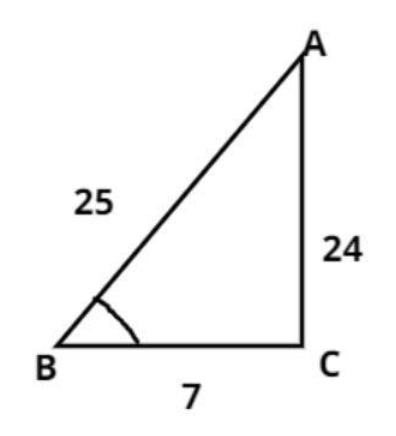Courses
Courses for Kids
Free study material
Offline Centres
MoreLast updated date: 30th Nov 2023
Total views: 384k
Views today: 4.84k

# If $\tan \theta = \dfrac{{24}}{7}$, then find the value of $\sin \theta + \cos \theta$.Verified
384k+ views
Hint: - Use Pythagoras theorem, $\left[ {{{\left( {{\text{Perpendicular}}} \right)}^2} + {{\left( {{\text{Base}}} \right)}^2} = {{\left( {{\text{Hypotenuse}}} \right)}^2}} \right]$Let, ABC is a right angle triangle, in which $\angle {\text{C}} = {90^0}$, and $\angle {\text{B = }}\theta$
Given:
$\tan \theta = \dfrac{{24}}{7}$
Therefore in $\Delta {\text{ABC, }}\tan \theta = \dfrac{{{\text{AC}}}}{{{\text{BC}}}}$
$\Rightarrow {\text{AC = 24, and BC = 7}}$
Apply Pythagoras Theorem in $\Delta {\text{ABC}}$
$\left[ {{{\left( {{\text{Perpendicular}}} \right)}^2} + {{\left( {{\text{Base}}} \right)}^2} = {{\left( {{\text{Hypotenuse}}} \right)}^2}} \right]$
$\Rightarrow {\text{A}}{{\text{B}}^2} = {\text{A}}{{\text{C}}^2} + {\text{B}}{{\text{C}}^2} \\ \Rightarrow {\text{A}}{{\text{B}}^2} = {24^2} + {7^2} = 625 = {\left( {25} \right)^2} \\ \Rightarrow {\text{AB = 25}} \\$
Therefore in $\Delta {\text{ABC}}$, $\sin \theta = \dfrac{{{\text{AC}}}}{{{\text{AB}}}}$ and $\cos \theta = \dfrac{{{\text{BC}}}}{{{\text{AB}}}}$
So, the value of $\sin \theta + {\text{ }}\cos \theta = \dfrac{{24}}{{25}} + \dfrac{7}{{25}} = \dfrac{{31}}{{25}}$
So, this is the required answer.

Note- In such types of questions always apply Pythagoras Theorem which is stated above, then calculate the value of $\sin \theta$ which is perpendicular divided by hypotenuse, then calculate the value of $\cos \theta$ which is base divided by hypotenuse, then add these values we will get the required answer.